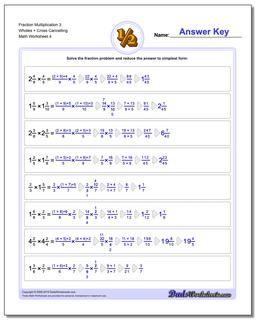# Math Worksheets: Fraction Multiplication: Fraction Multiplication: Fraction Multiplication 3 Wholes + Cross Cancelling (Fourth Worksheet)## Fraction Multiplication 3 Wholes + Cross Cancelling (Fourth Worksheet)

PropertyValue
DescriptionFraction Multiplication 3 Wholes + Cross Cancelling: Basic fraction multiplication with whole parts and cross-cancelling (Fourth Worksheet)
Resource TypeWorksheet